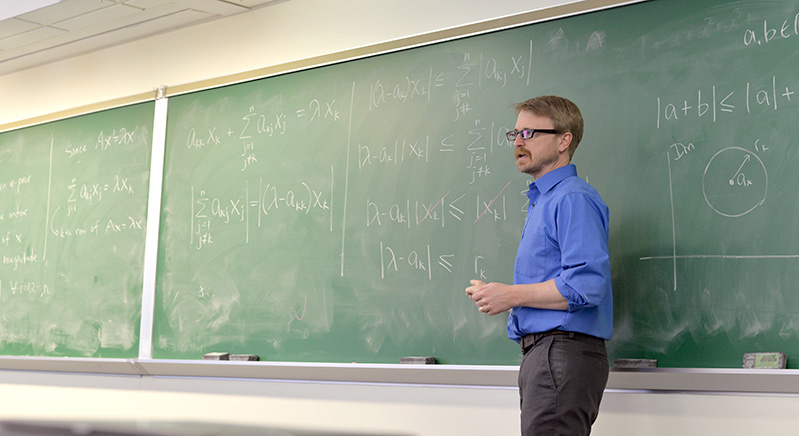# Math - Mathematics

Overview
WabashX Course Catalog registrar Phi Beta Kappa Research Teach At Wabash LibraryMathematics is the study of patterns and the logical connections between them. The patterns can be numerical, algebraic, or geometric. The logical connections are typically computations and proofs. When the patterns come from the real world, we get applied mathematics. The logical connections might then take the form of a differential equation that predicts how a disease outbreak will unfold, a statistical model that allows an actuary to assess risks, or a geometric algorithm that displays a three-dimensional object on a flat computer screen. When the patterns come from our collective imaginations, we get the myriad sub-disciplines of pure mathematics: real analysis, abstract algebra, topology, non-Euclidean geometry, probability, and many others.

The mathematics program has the following goals:

• To give all students who take mathematics courses a sense of the nature of mathematics and its place in society;
• To give our mathematics majors and minors an understanding of mathematics, its nature and uses; to prepare students to become effective users of mathematics in their careers;
• To prepare future high school teachers of mathematics;
• To give our students interested in continuing to graduate study in mathematics, statistics, or computer science an adequate preparation to succeed in that study.
• To prepare students to excel in their majors. This includes students in distribution courses, mathematics and computer science minors, and students with double majors, who will gain deeper insights into their other majors.

The mathematics major can tailor upper-level courses to his interests (including pure mathematics, applied mathematics and statistics) and career goals (including actuarial science, computer science and secondary education).